# Owner of the company

The owner of the company with extensive administrative activity wants to sell the obsolete 3 machines at the price of CZK 1000 per 1 machine with a six-month warranty period. If the machine breaks down during this period, the owner will return the employees money and deliver 100 CZK for the removal of unused machines. Determine the most number of machines that the owner should sell off, assuming a ten percent failure rate and if he wish to make the lot of money.

Result

n =  3

Leave us a comment of example and its solution (i.e. if it is still somewhat unclear...):

Showing 0 comments:Be the first to comment!#### To solve this example are needed these knowledge from mathematics:

Would you like to compute count of combinations?

## Next similar examples:

1. Normal distribution GPA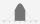The average GPA is 2.78 with a standard deviation of 4.5. What are students in the bottom the 20% having what GPA?
2. Balls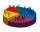We have n identical balls (numbered 1-n) is selected without replacement. Determine 1) The probability that at least one tensile strength number coincides with the number of balls? 2) Determine the mean and variance of the number of balls, which coincides.
3. Hearts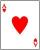5 cards are chosen from a standard deck of 52 playing cards (13 hearts) with replacement. What is the probability of choosing 5 hearts in a row?
4. BallsFrom the urn in which are 7 white balls and 17 red, gradually drag 3-times without replacement. What is the probability that pulls balls are in order: red red red?
5. Theorem proveWe want to prove the sentense: If the natural number n is divisible by six, then n is divisible by three. From what assumption we started?
6. Class - boys and girls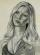In the class are 60% boys and 40% girls. Long hair has 10% boys and 80% girls. a) What is the probability that a randomly chosen person has long hair? b) The selected person has long hair. What is the probability that it is a girl?
7. One greenIn the container are 45 white and 15 balls. We randomly select 5 balls. What is the probability that it will be a maximum one green?
8. CardsSuppose that are three cards in the hats. One is red on both sides, one of which is black on both sides, and a third one side red and the second black. We are pulled out of a hat randomly one card and we see that one side of it is red. What is the probabi
9. CardsThe player gets 8 cards of 32. What is the probability that it gets a) all 4 aces b) at least 1 ace
10. AsymptoteWhat is the vertical asymptote of ?
11. Lottery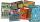Fernando has two lottery tickets each from other lottery. In the first is 973 000 lottery tickets from them wins 687 000, the second has 1425 000 lottery tickets from them wins 1425 000 tickets. What is the probability that at least one Fernando's ticket w
12. Quadratic function 2Which of the points belong function f:y= 2x2- 3x + 1 : A(-2, 15) B (3,10) C (1,4)
13. Box and whisker plotConstruct a box and whisker plot for the given data. 56, 32, 54, 32, 23, 67, 23, 45, 12, 32, 34, 24, 36, 47, 19, 43
14. Quadratic equationFind the roots of the quadratic equation: 3x2-4x + (-4) = 0.
15. CalculationHow much is sum of square root of six and the square root of 225?
16. TrinityHow many different triads can be selected from the group 43 students?
17. Derivation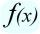Exists a function whose derivation is the same function?